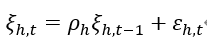# Ask for help: A DSGE model with housing market

I’m new to learning DSGE model to solve housing price problems, but I find it’s very hard for me to create a model, probably because I don’t know how to “transform” the first order condition to my dynare program . So can you give me some suggestion about introduction book or papers？It will be very helpful, Thank you!

What do you mean with “transform”?

Thank you for reply me! For example, an logAR(1) process like，I don’t know how to linearize it.

That one would simply be linearized to obtain
\hat \xi_{h,t}=\rho_h\hat \xi_{h,t-1}+\varepsilon_{h,t}

Thank you !What make me confused is the linearization of AR（1） process likealso has the same form as logAR（1） process. What is the difference between them?

Essentially, you are approximating ln(1+x)\approx x for x\approx0. Hence, the nonlinear logarithm is linearly approximated. The rest of the equation is already linear.

1 Like